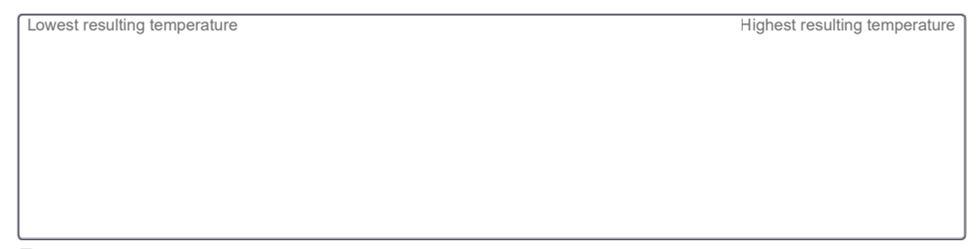# Problem: Suppose 1 kg each of water (4.19 J/(g⋅°C)), brick (0.90 J/(g⋅°C)), iron (0.46 J/(g⋅°C)), and olive oil (1.79 J/(g⋅°C)) were held at the same initial temperature and heated for an equivalent amount of time. Indicate their relative final temperatures from lowest (left) to highest (right). Assume no heat is lost to the surroundings. You can use the Intro tab of the PhET to help visualize the temperature changes. (Assume that the mass of the water, iron, and olive oil is 1 kg each and that the mass of the brick is 0.5 kg.) Rank from lowest resulting temperature to highest resulting temperature. To rank items as equivalent, overlap them.Water, Olive oil, Brick, IronHeat capacity refers to how much energy a material can absorb with respect to changes in average kinetic energy, and specific heat capacity quantifies the exact amount of energy it takes to raise the temperature of 1 g of a material by 1 ∘C (depending on the units, which can sometimes refer to the moles of material). Heat capacity refers to the temperature changes in the same state of matter, whereas the per unit energies required to melt and boil a substance are called the enthalpies of fusion and vaporization, respectively. The relationship between heat and temperature change is described by the following equation: q = mcsΔT where q is the heat absorbed or lost, m is the mass, cs is the specific heat, and ΔT is the change in temperature.

###### FREE Expert Solution
79% (27 ratings)View Complete Written Solution
###### Problem Details

Suppose 1 kg each of water (4.19 J/(g⋅°C)), brick (0.90 J/(g⋅°C)), iron (0.46 J/(g⋅°C)), and olive oil (1.79 J/(g⋅°C)) were held at the same initial temperature and heated for an equivalent amount of time. Indicate their relative final temperatures from lowest (left) to highest (right). Assume no heat is lost to the surroundings. You can use the Intro tab of the PhET to help visualize the temperature changes. (Assume that the mass of the water, iron, and olive oil is 1 kg each and that the mass of the brick is 0.5 kg.) Rank from lowest resulting temperature to highest resulting temperature. To rank items as equivalent, overlap them.

Water, Olive oil, Brick, IronHeat capacity refers to how much energy a material can absorb with respect to changes in average kinetic energy, and specific heat capacity quantifies the exact amount of energy it takes to raise the temperature of 1 g of a material by 1 ∘C (depending on the units, which can sometimes refer to the moles of material). Heat capacity refers to the temperature changes in the same state of matter, whereas the per unit energies required to melt and boil a substance are called the enthalpies of fusion and vaporization, respectively. The relationship between heat and temperature change is described by the following equation:

where q is the heat absorbed or lost, m is the mass, cs is the specific heat, and ΔT is the change in temperature.